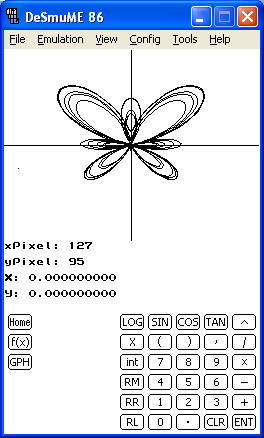graphDS is a graphing calculator application designed for the Nintendo DS. Currently, the program is capable of evaluating mathematical expressions and Riemann sums as well as simultaneiously graphing up to four Cartesian, parametric, or polar functions.Changes:

-Evaluates mathematical expressions… including those with exponents, trig, and logarithmic functions
-Graphs Cartesian, parametric, and polar equations
-Catalog with many different functions
-Evaluates numerical derivatives and integrals, as well as Riemann sums

http://robertson.heliohost.org/graphDS.html